﻿ Predictive Modeling of <i>Toxoplasma Gondii</i> Activity of a Series of Substituted Imidazole-Thiosemicarbazides Using Quantum DescriptorsPublications are Open
Access in this journal
Article Versions
Export Article
• Normal Style
• MLA Style
• APA Style
• Chicago Style
Research Article
Open Access Peer-reviewed

### Predictive Modeling of Toxoplasma Gondii Activity of a Series of Substituted Imidazole-Thiosemicarbazides Using Quantum Descriptors

Sopi Thomas Affi, Bafétigué Ouattara, Georges Stéphane Dembélé, Mamadou Guy-Richard Koné , Nahossé Ziao
Physics and Materials Chemistry. 2021, 7(1), 1-13. DOI: 10.12691/pmc-7-1-1
Received November 01, 2021; Revised December 03, 2021; Accepted December 12, 2021

### Abstract

Quantitative Structure Activity Relationship (QSAR) study of Toxoplasma gondii was done on a series of twenty-five (25) imidazole-thiosemicarbazide molecules. In order to obtain molecular descriptors all these molecules were optimized at B3LYP/LanL2DZ level. This study was performed using the linear multiple regression (MLR), nonlinear regression (MNLR) and artificial neural network (ANN) methods. These statistical methods allow to find three (3) quantitative models. Quantum descriptors which such as energy gap (ΔE), dipole moment (μ), enthalpy of formation (ΔfH), bond length (D(C-S)) and lipophilicity (Logp) were used in models elaboration. Among obtained models, RNA model has much better predictive ability than other models with R2 = 0.9291 and RMCE = 0.00023. A decrease in energy gap (ΔE) which is the main descriptor could significantly improve the Toxoplasma gondii IC50 inhibitory concentration of substituted imidazole-thiosemicarbazide analogues. Furthermore, the external validation test pIC50 theo/ pIC50 exp and the applicability domain from Cook's distance were verified.

### 1. Introduction

Toxoplasmosis is a disease which is caused by infection with a parasite called Toxoplasma gondii. It is usually transmitted to humans by domestic animals, especially cats, or by ingestion of meat which is not well cooked. Toxoplasma gondii affects about 30% of population around the world 1. This parasite causes severe disease in people with HIV/AIDS or in pregnant women creating congenital malformations 2, 3. In this context, Agata et al 4, 5 have synthesized and tested the substituted imidazole-thiosemicarbazides to fight against all infections caused by Toxoplasma gondii. Imidazole-thiosemicarbazides are convenient precursors that have been widely used in heterocyclic synthesis. Since a longtime studies on heterocyclic compounds have been an area of interest in medicinal chemistry. Indeed, these compounds and their derivatives have a wide spectrum of biological activities namely antibacterial 6, antifungal 7, anticonvulsant 8, antimicrobial 9, antitumor 10. IC50 inhibitory activity of imidazole-thiosemicarbazides was used as backbone of our study. The Quantitative Structure-Activity Relationship (QSAR) study, is the method that allows to correlate the molecular structure with a well determined effect such as biological activity or chemical reactivity. It is increasingly used to reduce the excessive number of experiments which are sometimes long, dangerous and costly in terms of time and money 11, 12. The present work is made in the perspective to establish a model which will be able to fight against the infection caused by Toxoplasma gondii. All this contributes to the reduction of drug production costs 13, 14 and contributes to the protection of environment. In general, the QSAR model is a function of one fifth (1/5) of the initial database.

The main objective of this work is to develop reliable models to explain and predict the IC50 antibacterial activity (median inhibitory concentration in μg/mL) of a series of twenty-five (25) substituted imidazole-thiosemicarbazide derivatives (Figure 1). These compounds were synthesized and tested by Agata et al 4, 5 for their biological activities.

• Figure 1. Molecular structure, code and inhibitory concentration (IC50) of the twenty-five (25) substituted imidazole-thiosemicarbazide derivatives

### 2. Materials and Methods

2.1. Computational Level of Theory

In order to predict the antibacterial activity of imidazole-thiosemicarbazides quantum chemical calculations were performed using Gaussian 09 software 15. DFT methods are generally known to generate a variety of molecular properties 16, 17, 18 in QSAR studies. These increase the predictability of QSAR models while reducing the computational time and implication of cost in new drug conception 19, 20. The B3LYP/LanL 2 DZ level of theory was used to determine the molecular descriptors. The modeling was done using three methods. The first method is multilinear and nonlinear regression (MNLR) regression which are implemented in Excel 21 and XLSTAT 22 spreadsheets. The third method is the artificial neuron method which is included in the JMP Pro software 23.

2.2. Used Molecular Descriptors

In order to develop our QSAR model, some theoretical descriptors were determined. In particular, the energy gap (ΔE), dipole moment (μ), enthalpy of formation (ΔfH), bond length (D(C-S)) and lipophilicity (Logp).

The energy gap represents the energy difference between the boundary orbitals namely HOMO and LUMO, it is a parameter that gives more information about reactivity and stability of a molecule. The interactions are more favored, when the energy gap (ΔE) between the HOMO and LUMO is smaller 24. They are thus stabilizing for the new chemical edifice formed 25. The energy gap (ΔE) is calculated from equation (1):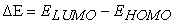(1)

The theory of boundary molecular orbitals allows us to analyze the reactivity of molecules which is made in terms of the interactions of reactants molecular orbitals 24. But energies likeetcan be used to calculate many other parameters.

Lipophilicity expresses the affinity of a molecule for a lipidic environment such as oil, cell membrane, lipidic solvent 26. This physico-chemical parameter is commonly measured by the distribution of neutral ou soluble molecule, between water and another immiscible solvent like n-octanol (or octan-1-ol) which is generally used 26, 27, 28, 29. Lipophilicity is evaluated from the value of logP. LogP. is equal to the logarithm of concentrations ratio of the studied substance in octanol and in water logP = log (Coct/Water). Indeed, positive and very high value of logP expresses the fact that the considered molecule is much more soluble in octanol than in water, which reflects its lipophilic character, Conversely, logP with negative value means that the considered molecule is hydrophilic. When logP is nul, it means that the molecule has the same solubility level in the two solvents. In this work, Chemsketch software 30 was used to determine the different values of logp. In practice we express the lipophilicity by the decimal logarithm of the partition coefficient logP. Thus :

If logP > 0; then P>1, the molecule is lipophilic. It is soluble in the lipidic phase so the molecule is not polar.

If logP < 0 then P<1, the molecule is hydrophilic. It is soluble in water so the molecule is then polar.

Another parameter related to the distribution of charges, is the dipole moment. This parameter is based on the existence of electrostatic dipoles. It is a global distribution of electric charges in a molecular system, so that the barycenter of positive charges does not coincide with that of negative charges. The dipole moment is a vector quantity. For a distribution of chargesat distance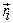each, the total dipole moment is established as follows: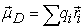(2)

The dipole moment makes it possible to describe the global polarity as well as the existence of interactions of molecular systems such as Van der Waals forces, and also to predict their solubility in polar solvents. The dipole moment is an important property that gives an idea of the reactivity of the molecule 31. Furthermore, it indicates the stability of a molecule in water. Thus, a high dipole moment will reflect a low solubility in organic solvents and a high solubility in water 37, 38.

The standard enthalpy of formation at temperature T of a chemical compound is the difference in enthalpy involved in the formation of one mole of this compound from simple, pure bodies, taken in the standard state and stable at the temperature considered T. The enthalpy of formation is calculated through the following formulas proposed by Otchersky et al 15:(3)(4)

with:(5): Atomization energy;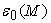: Total energy of the molecule;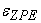: Zero point energy of the molecule;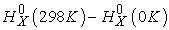: Enthalpy corrections of the atomic elements. These values are included in the table of Janaf 32.: Enthalpy correction of the molecule: Enthalpy of thermal correction.

The geometric descriptor used is the bond length d(C-S) in Armstrong (A°) (Figure 2). This descriptor is illustrated in the figure below around the imidazole-thiosemicarbazide ring.

• Figure 2. Geometric descriptor of the substituted imidazole-thiosemicarbazide derivatives: the bond length (D(C-S)) in Armstrong (A°)

For all the descriptors studied, the analysis of the bivariate data, i.e. the calculation of the partial correlation coefficient between each pair of the set of descriptors is less than 0.70 (aij < 0.70), which means that these different descriptors are independent of each other 33, 34. To better understand the interdependence of the descriptors used, we present the partial correlation coefficient values aij of these descriptors in Table 1.

The partial correlation coefficients aij contained in Table 1 between the descriptor pairs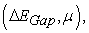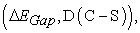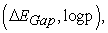(µ, ΔfH), (µ, D(C-S)), (µ, logp), (ΔfH, D(C-S)), (ΔfH logp), and (D(C-S), logp) are less than 0.95 (aij < 0.70). This demonstrates the independence of the descriptors used to develop the models.

2.3. Estimation of the Predictive Capacity of a QSAR Model

The quality of a model is determined on the basis of various statistical criteria of analysis, including the coefficient of determination R2, the standard deviation RMCE, the correlation coefficients of the cross-validationand Fischer F. R2, S and F relate to the fit of the calculated and experimental values. They describe the predictive ability within the limits of the model, and allow us to estimate the accuracy of the calculated values on the test set 35, 36. As for the cross-validation coefficient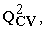it provides information on the predictive power of the model. This predictive power is called "internal" because it is calculated from the structures used to build the model. The correlation coefficient gives an evaluation of the dispersion of the theoretical values around the experimental values. The quality of the modeling is better when the points are close to the fitting line 37. The fit of the points to this line can be evaluated by the coefficient of determination.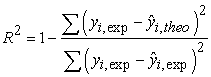(6)

Where:: Experimental value of anticancer activity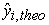: Theoretical value of anticancer activity and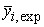: Average value of the experimental values of the anticancer activity.

The closer the value will be to 1 the more correlated the theoretical and experimental values are

On the other hand, the varianceis determined by relation 7:(7)

Where k is the number of independent variables (descriptors), n is the number of molecules in the test or training set and n-k-1 is the degree of freedom.

The standard deviation or RMCE is another statistical indicator used. It allows to evaluate the reliability and the precision of a model:(8)

The Fisher F test is also used to measure the level of statistical significance of the model, i.e. the quality of the choice of descriptors making up the model.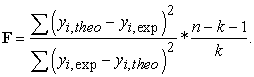(9)

The coefficient of determination of the cross-validationallows to evaluate the accuracy of the prediction on the test set. It is calculated using the following relation: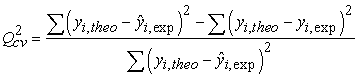(10)
2.4. Criterion of Acceptance of a Model

The performance of a mathematical model, for Eriksson et al 38, is characterized by a value of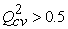for a satisfactory model when for the excellent model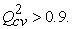According to them, given a test set, a model will perform well if the acceptance criterionis met.

2.5. Statistical Analysis
2.5.1. Principal Component Analysis (PCA)

Principal Component Analysis (PCA) is a data analysis tool that allows to explain the structure of correlations or covariances using linear combinations of the original data. Its use allows the interpretation of data in a reduced space 39. It has been used to assess the relationships between the different variables measured, but above all to access their structure in order to group them by zone. The grouping by zone thus meets the objective of this approach, which is to correlate the classes of physico-chemical descriptors obtained at the sampling stations.

2.5.2. Ascending Hierarchical Classification (AHC)

The purpose of the Ascending Hierarchical Classification (AHC) is to partition a set of individuals into homogeneous classes (an individual is an observation and, in our case, these are samples) 40. It organizes the individuals, defined by a certain number of variables and modalities, by grouping them hierarchically on a dendrogram. It aggregates those that are most similar to each other using measures of dissimilarity or distance between individuals to form classes. It is performed using data from individuals and variables. AMP allowed for a typology of samples based on energy gap (ΔE), dipole moment (μ), enthalpy of formation (ΔfH), bond length (D(C-S)) and lipophilicity (Logp).

2.5.3. Multiple Linear and Non-Linear Regressions (MLR and NLMR)

The statistical technique of multiple linear regression (MLR) is used to study the relationship between a dependent variable (Property) and several independent variables (descriptors). This statistical method minimizes the differences between the actual and predicted values. It was also used to select the descriptors used as input parameters in the multiple nonlinear regression (MNLR). As for the multiple non-linear regression (MNLR) analysis, it also allows to improve the structure-property relationship in order to quantitatively evaluate the property. It is the most common tool for studying multidimensional data. It is based on the following pre-programmed functions of XLSTAT: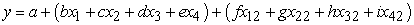(11)

Où a, b, c, d,... are the parameters and, x1, x2, x3, x4,... are the variables.

2.5.4. Artificial Neuron Network (ANN)

Artificial neurons are an inspiration of the human biological neuron. To this end, they are made up of cells or neurons linked together by connections that allow them to send and receive signals from other cells. These neurons are mathematical models made up of several neurons, arranged in different layers. Generally, the network consists of three layers; an input layer, a hidden layer and an output layer, connected through a complex network 41, 42. The most commonly used networks are the Multi-Layer Perceptrons (MLP) whose neurons are generally arranged on layers 43. In this work, the artificial neural network was obtained using the 5-3-1 multilayer perceptron network, i.e., the network consists of five (5) neurons in the input layer, three (3) neurons in the hidden layer and one (1) neuron in the output layer. The output layer consists of a sigmoid function. The architecture of the applied ANN models is presented in (Figure 3).

2.6. Acceptance Criteria of a QSAR Model

The twenty-five (25) molecules used in this study have Inhibitory Concentration (IC) ranging from 10.3 to 113.45 μg/mL. The median inhibitory concentration (IC50) is a measure of the effectiveness of a given compound in inhibiting a specific biological or biochemical function. Biological data are usually expressed as the opposite of the decimal-based logarithm of activity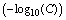to obtain better mathematical values when structures are biologically active 44, 45. The antibacterial activity will be expressed by the antibacterial potential pIC50 defined by equation (12):(12)

Where M is the molecular weight of the compound in g/mol and IC is the Inhibitory Concentration in μg/mL.

• Figure 3. Diagram of the structure of a multilayer perceptron
2.7. Domain of Applicability (DA)

The domain of applicability of a QSAR model is the physicochemical, structural, or biological space in which the model equation is applicable to make predictions for new compounds 46. It corresponds to the region of chemical space including the compounds in the training set and similar compounds, which are close in the same space 47. Indeed, the model, which is built on the basis of a limited number of compounds, by relevant descriptors, chosen among many others, cannot be a universal tool to predict the activity of any other molecule with confidence. It appears necessary, even mandatory, to determine the DA of any QSAR model. This is recommended by the Organization for Economic Cooperation and Development (OECD) in the development of a QSAR model 48. There are several methods for determining the domain of applicability of a model 47. Of these, the approach used in this work is the Cook distance. The Cook distance measures the effect of deleting a data item. Data with large residuals (outliers) and/or high leverage can distort the outcome and accuracy of a regression. The threshold Cook distance is determined by the following expression 49, 50.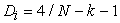(13)

With N: the number of observations, k: the number of descriptors defined by the model.

### 3. Results and Discussion

All the values of the physico-chemical descriptors of nineteen (19) compounds of the test set and six (6) other compounds of the validation set are presented in Table 2.

3.1. Typology of Physicochemical Descriptors of Imidazole-Thiosemicarbazide Derivatives

The correlation circle (Figure 4), the Cartesian diagrams according to F1 and F2 (Figure 5) and the dendrogram of the compounds are shown below.

• Figure 4. Correlation circle of descriptors descriptors according to F1 x F2
• Figure 5. Cartesian diagram according to F1 and F2: correlation between the physicochemical descriptors used and the inhibitory concentration IC50
• Figure 6. Dendrogram of molecules

PCA data matrix groups the mean values of five (5) variables representing physicochemical descriptors and twenty-five (25) molecules. The resulting matrix provides information on the negative or positive correlation between the variables. The examination of the community circle in Figure 4 associated with the analysis of the factorial structure of the PCA, indicate that two (02) principal components represented by F1 which corresponds to 39.18% of the explained variance and F2 with 25.97% of the explained variance. The two factors F1 and F2 totaling 65.15% of the total variance are sufficient to interpret all the PCA data. Each variable is associated with its factorial weight. Thus, the energy gap (0.88) and the enthalpy of formation (0.70) are positively correlated to factor 1, while factor 2 is positively correlated to the dipole moment (0.45) and the bond length (D(C-S)) (0.64). It appears from this analysis that the F1 factor (39.18%) correlates better with the energy gap and the enthalpy of formation with very high coefficients.

Cartesian diagram in Figure 5 shows that compounds 1, 20, and 6 negatively correlated with factor 2 with an inhibitory potential pIC50. Molecules 14 and 17 are positively correlated with dipole moment μ(D) and factor 1. It must be said that compounds 18,13, 11, 12 and 21 are positively correlated with factor 1 and strongly correlated with distance D(C-S). The molecules 16, 19 and 4 are correlated to the energy gap ΔE and positively factor 1. The same is true for the molecules 15 and 5. The last group of compounds (2, 3, 6, 7, 8, 9, 10, 11, 12, 13, 18, 21, 22, 23, 24 and 25) correlate with logp and enthalpy of formation ΔfH. The latter classification is positively influenced with factor 2 and negatively with factor 1.

The illustration of the distribution of the compounds in the Cartesian diagram is also reflected in the dendrogram of the molecules (Figure 6). In Figure 6, the compounds are grouped into 4 classes (C1, C2, C3 and C4) characterizing the physicochemical descriptors. Thus, the class C1 (1, 6, and 20) which consists of three molecules. We have the class C2 composed of two(2) molecules (14 and 17) strongly influenced by the dipole moment μ(D) and the class C3 constituted by ten(10) molecules (4, 5, 11, 12, 13, 15, 16, 18, 19 and 21) influenced by the distance D(C-S). The last class C4 grouping the remaining ten(10) molecules (2, 3, 7, 8, 9, 10, 22, 23, 24 and 25) strongly correlated with the logp descriptors and the enthalpy of formation ΔfH.

3.2. Multilinear Regression Models (MLR) and Nonlinear Multiple Regression (NLMR)

In a model equation, the negative or positive sign of the coefficient of a descriptor reflects the proportionality effect between the evolution of the inhibitory concentration IC50 and this physicochemical parameter in the regression equation. Thus, the negative sign indicates that when the value of the descriptor is high, the inhibitory concentration IC50 decreases, whereas the positive sign reflects the opposite effect. The equations of the best obtained MLR and NLMR models, as well as the statistical indicators will be presented in Table 3 below. These statistical indicators will be followed by Figure 6 and Figure 7 presenting the fit line of the experimental and theoretical data of the pIC50 inhibitory potentials of the test (blue dots) and validation (red dots) sets of the model. It should be noted that these models were built using the same test and validation sets in Table 1.

Model MLR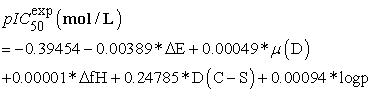Model NLMR• Figure 7. Regression line of the MLR model
• Figure 8. Regression line of the NLMR model

The negative correlation coefficient between the energy gap ΔE and the inhibitory potential pIC50 indicates that these two variables are inversely proportional i.e. decreasing the energy gap favors increasing the inhibitory concentration IC50 in both models. The significance of these models is shown by the high values of the Fischer F coefficient which are 85.794 and 137.07 for the MLR and NLMR models respectively. Moreover, the robustness of these models is reflected by the cross-validation correlation coefficient Q2cv which are also 0.8347 and 0.8897. These MLR and NLMR models are all acceptable because R2 - Q2cv =0.000<0.3. The regression lines established by the experimental and theoretical data of pIC50 inhibitory potential of the test and validation sets for MLR and NLMR models are shown in Figure 7 and Figure 8.

The external validation test was verified by calculating the pIC50 theo/ pIC50 exp ratio of the MLR and NLMR models. These values are confined in Table 4.

The values of the ratio pIC50 theo/ pIC50 exp of the model validation set tend to unity (Table 4) reflecting the good correlation between the theoretical and experimental pIC50 inhibitory potential of the observations. These models are therefore acceptable for the prediction of the inhibitory concentration IC50 of substituted imidazole-thiosemicarbazide derivatives.

Moreover, the low values of the standard error (RMCE) which are 0.00038 and 0.0004 for the MLR and NLMR models respectively attest to the good similarity between the predicted and experimental values (Figure 9 and Figure 10). These curves show a similar evolution of the data from these two models for the prediction of the inhibitory concentration IC50 of substituted imidazole-thiosemicarbazide derivatives despite some deviations recorded.

• Figure 9. Similarity curve of the experimental and predicted values of the MLR model
• Figure 10. Similarity curve of the experimental and predicted values of the NLMR model

The values of the pIC50 theo/ pIC50 exp ratio of the validation set that tend to unity (Table 3) reflect the good correlation between the theoretical and experimental inhibitory concentrations IC50 of the observations. Therefore, these models are acceptable for the prediction of the IC50 inhibitory concentration of substituted imidazole-thiosemicarbazide derivatives.

3.3. Artificial Neural Network (ANN)

The equation of the best model of the Artificial Neural Network obtained, as well as the statistical indicators will be proposed in Table 5 below. This equation will be followed by 3 other equations (X1, X2, X3) obtained from the hidden layer. These three equations from the hidden layer are hyperbolic tangent functions dependent on the molecular descriptors (ΔE, μD, ΔfH, D(C-S, logp).

The statistical indicators of the ANN model will be followed by Figure 11 presenting the fit line of the experimental and theoretical data of the pIC50 inhibitory potentials of the test and validation sets. Here these fitting lines of the test set and the validation set include twenty (20) and five (5) molecules respectively.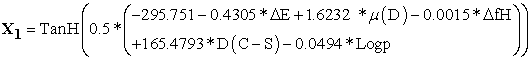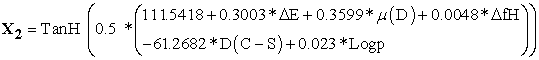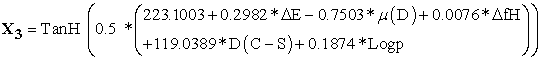• Figure 11. Graphs of observed values versus predicted values of test and validation sets

Of the three models, the model obtained by the statistical ANN method has a much better predictive capacity. However, since this model is a function of five physico-chemical descriptors, it is essential to determine the contribution of each one in the prediction of the inhibitory concentration IC50. Indeed, the knowledge of this contribution makes it possible to establish the order of priority of the various descriptors and to define the choice of the parameters to be optimized for the good prediction and comprehension of the inhibitory concentration IC50 of the substituted imidazole-thiosemicarbazide derivatives.

3.4. Analysis of the Contribution of the Descriptors

The contributions of the five physico-chemical descriptors in predicting the inhibitory concentration IC50 of substituted imidazole-thiosemicarbazide derivatives were illustrated by the normalized coefficients and shown by Figure 12.

• Figure 12. Contribution of descriptors in the models

According to the contribution of these descriptors, the energy gap (ΔE) displays the highest normalized coefficient (-2.2771) followed by the enthalpy of formation (ΔfH) with 1.8944 and the dipole moment (μD) holds the lowest coefficient (0.6279) compared to the other descriptors. It should be noted that the energy gap (ΔE) is the most influential physicochemical descriptor. Thus, to improve the inhibitory concentration IC50 in the synthesis of new substituted imidazole-thiosemicarbazide derivatives, the energy gap (ΔE) must be played to the maximum extent.

3.5. Domain of Applicability of the Model

Here the analysis of the applicability domain in our study being based on the determination of the Cook threshold distance. This parameter was evaluated at the level of the test set.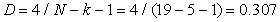(14)

The cook distances of our molecules are listed in Table 6 and are illustrated in Figure 12.

Here, we observe a large influence of compounds 8, 16 and 17 on the parameter estimation and predictions. To a lesser extent, the molecules 2, 3, 6 and 7. Compounds 8, 16 and 17 are considered to be outside the applicability. These erroneous predictions could probably be attributed to erroneous experimental data or the structure of these outliers.

• Figure 12. Cook's distance from the test set

### 4. Conclusion

At the end of this study we were able to show a relationship between the inhibitory concentration IC50 of Toxoplasma gondii and the physicochemical descriptors of imidazole-thiosemicarbazides. The descriptors energy gap (ΔE), dipole moment (μ), enthalpy of formation (ΔfH), bond length (D(C-S)), and lipophilicity (Logp) help explain and predict the antiparasitic activity of imidazole-thiosemicarbazides. Statistical methods such as principal component analysis (PCA), Ascending Hierarchical Classification (AHC), multilinear and nonlinear regression and artificial neuron method were employed. The study of the robustness of the three (3) models (MLR, NLMR and ANN) constructed shows a good predictive capacity. Moreover, compared to the MLR and NLMR models, the ANN model (R2 =0.9291; RMCE = 0.00023) is better and is an effective tool for predicting pest activity. The external validation test showed a good correlation between the theoretical and experimental pIC50 inhibitory potential of the observations. This translates that these models are therefore acceptable for the prediction of the inhibitory concentration IC50 of substituted imidazole-thiosemicarbazide derivatives. It is apparent from the contribution of the descriptors that a decrease in the energy gap (ΔE) could promote a significant improvement in the Toxoplasma gondii IC50 inhibitory concentration of the substituted imidazole-thiosemicarbazide analogues.

### References

Published with license by Science and Education Publishing, Copyright © 2021 Sopi Thomas Affi, Bafétigué Ouattara, Georges Stéphane Dembélé, Mamadou Guy-Richard Koné and Nahossé ZiaoThis work is licensed under a Creative Commons Attribution 4.0 International License. To view a copy of this license, visit http://creativecommons.org/licenses/by/4.0/